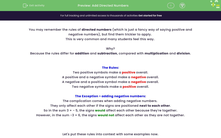### Comprehensive & curriculum aligned

In this worksheet, students will apply the rules related to positive and negative numbers to work out answers to addition sums.Key stage:  KS 4

Year:  GCSE

GCSE Subjects:   Maths

GCSE Boards:   Pearson Edexcel, OCR, Eduqas, AQA,

Curriculum topic:   Number, Number Operations and Integers

Curriculum subtopic:   Structure and Calculation Calculations with Integers

Difficulty level:#### Worksheet Overview

You may remember the rules of directed numbers (which is just a fancy way of saying positive and negative numbers), but find them trickier to apply.

This is very common and many students feel this way.

Why?

Because the rules differ for addition and subtraction, compared with multiplication and division.

The Rules:

Two positive symbols make a positive overall.

A positive and a negative symbol make a negative overall.

A negative and a positive symbol make a negative overall.

Two negative symbols make a positive overall.​

The Exception > adding negative numbers:

The complication comes when adding negative numbers.

They only affect each other if the signs are positioned next to each other.

So in the sum 3 + - 5, the signs would affect each other because they're together.

However, in the sum -3 + 6, the signs would not affect each other as they are not together.

Let's put these rules into context with some examples now.

e.g. Work out the value of: 3 + - 5

In this question we have a + and a - together, so they will affect each other.

They will become a negative overall.

This means we can rewrite the sum as: 3 - 5

As 5 is larger than 3, our answer will be less than 0 and a negative number.

If we picture a number line and start at 3, we then need to move 5 to the left to reach -2

e.g. Work out the value of: - 2 + - 4

In this question we have a + and a - together, so they will affect each other.

They will become a negative overall.

This means we can rewrite the sum as: - 2 - 4

If we picture a number line and start at -2, we then need to move 4 to the left to reach -6

In this activity, you will apply the rules related to positive and negative numbers to work out answers to addition sums.

### What is EdPlace?

We're your National Curriculum aligned online education content provider helping each child succeed in English, maths and science from year 1 to GCSE. With an EdPlace account you’ll be able to track and measure progress, helping each child achieve their best. We build confidence and attainment by personalising each child’s learning at a level that suits them.

Get started